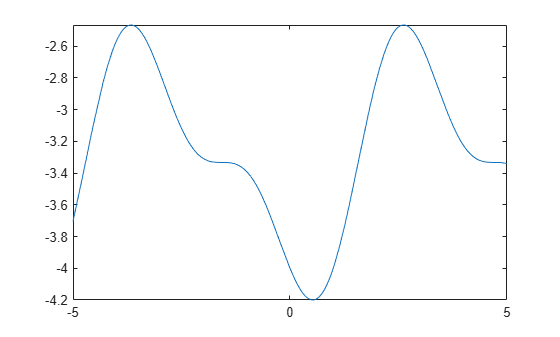equationsToMatrix

Convert linear equations to matrix form

Description

example

[A,b] = equationsToMatrix(eqns) converts equations eqns to matrix form. eqns must be a linear system of equations in all variables that symvar finds in eqns.

example

[A,b] = equationsToMatrix(eqns,vars) converts eqns to matrix form, where eqns must be linear in vars.

example

A = equationsToMatrix(___) returns only the coefficient matrix of the system of equations.

Examples

collapse all

Convert a system of linear equations to matrix form. equationsToMatrix automatically detects the variables in the equations by using symvar. The returned coefficient matrix follows the variable order determined by symvar.

syms x y z
eqns = [x+y-2*z == 0,
x+y+z == 1,
2*y-z == -5];
[A,b] = equationsToMatrix(eqns)
A =

$\left(\begin{array}{ccc}1& 1& -2\\ 1& 1& 1\\ 0& 2& -1\end{array}\right)$

b =

$\left(\begin{array}{c}0\\ 1\\ -5\end{array}\right)$

vars = symvar(eqns)
vars = $\left(\begin{array}{ccc}x& y& z\end{array}\right)$

You can change the arrangement of the coefficient matrix by specifying other variable order.

vars = [x,z,y];
[A,b] = equationsToMatrix(eqns,vars)
A =

$\left(\begin{array}{ccc}1& -2& 1\\ 1& 1& 1\\ 0& -1& 2\end{array}\right)$

b =

$\left(\begin{array}{c}0\\ 1\\ -5\end{array}\right)$

Convert a linear system of equations to the matrix form by specifying independent variables. This is useful when the equation are only linear in some variables.

For this system, specify the variables as [s t] because the system is not linear in r.

syms r s t
eqns = [s-2*t+r^2 == -1
3*s-t == 10];
vars = [s t];
[A,b] = equationsToMatrix(eqns,vars)
A =

$\left(\begin{array}{cc}1& -2\\ 3& -1\end{array}\right)$

b =

$\left(\begin{array}{c}-{r}^{2}-1\\ 10\end{array}\right)$

Return only the coefficient matrix of the equations by specifying a single output argument.

syms x y z
eqns = [x+y-2*z == 0,
x+y+z   == 1,
2*y-z   == -5];
vars = [x y z];
A = equationsToMatrix(eqns,vars)
A =

$\left(\begin{array}{ccc}1& 1& -2\\ 1& 1& 1\\ 0& 2& -1\end{array}\right)$

Consider the following system of linear equations that are functions of time:

$\begin{array}{l}2x\left(t\right)+y\left(t\right)+z\left(t\right)=2u\left(t\right)\\ -x\left(t\right)+y\left(t\right)-z\left(t\right)=v\left(t\right)\\ x\left(t\right)+2y\left(t\right)+3z\left(t\right)=-10\end{array}$

Declare the system of equations.

syms x(t) y(t) z(t) u(t) v(t)
eqn1 = 2*x + y + z == 2*u;
eqn2 = -x + y - z == v;
eqn3 = x + 2*y + 3*z == -10;
eqn = [eqn1; eqn2; eqn3]
eqn(t) =

$\left(\begin{array}{c}2 x\left(t\right)+y\left(t\right)+z\left(t\right)=2 u\left(t\right)\\ y\left(t\right)-x\left(t\right)-z\left(t\right)=v\left(t\right)\\ x\left(t\right)+2 y\left(t\right)+3 z\left(t\right)=-10\end{array}\right)$

Specify the independent variables $x\left(t\right)$, $y\left(t\right)$, and $z\left(t\right)$ in the equations as a symbolic vector vars. Use the equationsToMatrix function to convert the system of equations into the matrix form.

vars = [x(t); y(t); z(t)];
[A,b] = equationsToMatrix(eqn,vars)
A =

$\left(\begin{array}{ccc}2& 1& 1\\ -1& 1& -1\\ 1& 2& 3\end{array}\right)$

b =

$\left(\begin{array}{c}2 u\left(t\right)\\ v\left(t\right)\\ -10\end{array}\right)$

Solve the matrix form of the equations using the linsolve function.

X = linsolve(A,b)
X =

$\left(\begin{array}{c}\frac{10 u\left(t\right)}{9}-\frac{v\left(t\right)}{9}+\frac{20}{9}\\ \frac{4 u\left(t\right)}{9}+\frac{5 v\left(t\right)}{9}-\frac{10}{9}\\ -\frac{2 u\left(t\right)}{3}-\frac{v\left(t\right)}{3}-\frac{10}{3}\end{array}\right)$

Evaluate the $z\left(t\right)$ solution for the functions $u\left(t\right)=\mathrm{cos}\left(t\right)$ and $v\left(t\right)=\mathrm{sin}\left(2t\right)$. Plot the $z\left(t\right)$ solution.

zSol = subs(X(3),[u(t) v(t)],[cos(t) sin(2*t)])
zSol =

$-\frac{\mathrm{sin}\left(2 t\right)}{3}-\frac{2 \mathrm{cos}\left(t\right)}{3}-\frac{10}{3}$

fplot(zSol)Input Arguments

collapse all

Linear equations, specified as a vector of symbolic equations or expressions. Symbolic equations are defined by using the == operator, such as x + y == 1. For symbolic expressions, equationsToMatrix assumes that the right side is 0.

Equations must be linear in terms of vars.

Independent variables in eqns, specified as a vector of symbolic variables or symbolic functions.

Output Arguments

collapse all

Coefficient matrix of the system of linear equations, specified as a symbolic matrix.

Vector containing the right sides of equations, specified as a symbolic matrix.

collapse all

Matrix Representation of System of Linear Equations

A system of linear equations

$\begin{array}{l}{a}_{11}{x}_{1}+{a}_{12}{x}_{2}+\dots +{a}_{1n}{x}_{n}={b}_{1}\\ {a}_{21}{x}_{1}+{a}_{22}{x}_{2}+\dots +{a}_{2n}{x}_{n}={b}_{2}\\ \cdots \\ {a}_{m1}{x}_{1}+{a}_{m2}{x}_{2}+\dots +{a}_{mn}{x}_{n}={b}_{m}\end{array}$

can be represented as the matrix equation $A\cdot \stackrel{\to }{x}=\stackrel{\to }{b}$. Here, A is the coefficient matrix.

$A=\left(\begin{array}{ccc}{a}_{11}& \dots & {a}_{1n}\\ ⋮& \ddots & ⋮\\ {a}_{m1}& \cdots & {a}_{mn}\end{array}\right)$

$\stackrel{\to }{b}$ is the vector containing the right sides of equations.

$\stackrel{\to }{b}=\left(\begin{array}{c}{b}_{1}\\ ⋮\\ {b}_{m}\end{array}\right)$